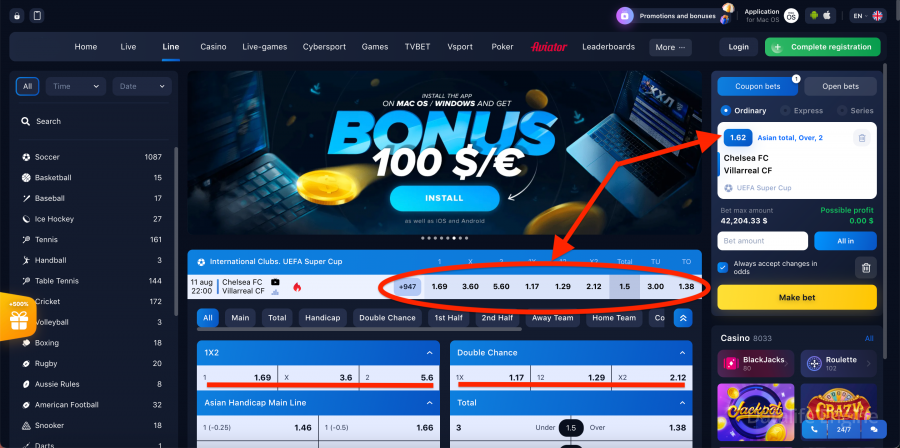# Odds in 1win bookmaker company

## Types of coefficients and methods of their calculation

There are three main types of odds:

• Decimal (European);
• Fractional (English);
• American.

Let's take a closer look at each of them. If you want to be “in the know”, study these basic odds definitions with examples, and you can easily calculate the odds yourself and place your bet correctly.### Decimal (European)

The simplest and most familiar one for perception. It looks like this - 1.58, 5.00, 2.45, etc. The winning amount in this case is determined by multiplying the deposit by the coefficient. For example: k = 2.5, deposit 1000, winning will be 2500.

### Fractional (English)

They look like this: 6/1, 4/2, 3/2, etc. The numerator is the amount of possible winnings, and the denominator is the bet (the amount wagered on the event).

Fractional odds are more common abroad. To convert a fractional coefficient into the decimal we are accustomed to, we need to divide the fraction and add 1: 6/3 + 1 = 3.0 to it.

### American view

The format of American odds is completely different. Coefficients are represented by “+” positive or “-” negative integers. The “-” sign means that the profit in case of winning will be less than the bet itself. For example, k = -200.
The number 200 denotes the amount of the bet required to get a win of 100 units. Thus, if you bet \$ 200, you will receive another \$ 100 if you win on top.

The plus sign is a simpler number scheme and here it denotes the amount of your net winnings if you bet 100 units. That is, if k = + 300, by betting \$ 100, you will receive a profit of \$ 300 in case of winning. Which of these systems is more convenient?
In Russia, the first two types (decimal and fractional) are more common and they are used on the official website 1winThe latter type is popular with American bookmakers.